# Area under the ROC curve – assessing discrimination in logistic regression

In a previous post we looked at the popular Hosmer-Lemeshow test for logistic regression, which can be viewed as assessing whether the model is well calibrated. In this post we’ll look at one approach to assessing the discrimination of a fitted logistic model, via the receiver operating characteristic (ROC) curve.

Before discussing the ROC curve, first let’s consider the difference between calibration and discrimination, in the context of logistic regression. As in previous posts, I’ll assume that we have an outcome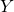, and covariates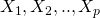. The logistic regression model assumes that: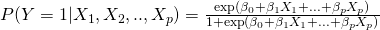The model parameters are the regression coefficients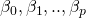, and these are usually estimated by the method of maximum likelihood.

Good calibration is not enough
For given values of the model covariates, we can obtain the predicted probability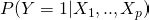. The model is said to be well calibrated if the observed risk matches the predicted risk (probability). That is, if we were to take a large group of observations which are assigned a value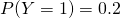, the proportion of these observations with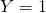ought to be close to 20%. If instead the observed proportion were 80%, we would probably agree that the model is not performing well – it is underestimating risk for these observations. The comparison between predicted probabilities and observed proportions is the basis for the Hosmer-Lemeshow test.

Should we be content to use a model so long as it is well calibrated? Unfortunately not. To see why, suppose we fit a model for our outcomebut without any covariates, i.e. the model: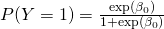This (null) model assigns every observation the same predicted probability, since it does not use any covariates. The estimate of the single parameter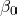will be the observed overall log odds of a positive outcome, such that the predicted value of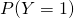will be identical to the proportion ofobservations in the dataset.

This (rather useless) model assigns every observation the same predicted probability. It will have good calibration – in future samples the observed proportion will be close to our estimated probability. However, the model isn’t really useful because it doesn’t discriminate between those observations at high risk and those at low risk. The situation is analogous to a weather forecaster who, every day, says the chance of rain tomorrow is 10%. This prediction might be well calibrated, but it doesn’t tell people whether it is more or less likely to rain on a given day, and so isn’t really a helpful forecast!

As well as being well calibrated, we would therefore like our model to have high discrimination ability. In the binary outcome context, this means that observations withought to be predicted high probabilities, and those with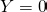ought to be assigned low probabilities. Such a model allows us to discriminate between low and high risk observations.

Sensitivity and specificity
To explain the ROC curve, we first recall the important notions of sensitivity and specificity of a test or prediction rule. The sensitivity is defined as the probability of the prediction rule or model predicting an observation as ‘positive’ given that in truth (). In words, the sensitivity is the proportion of truly positive observations which is classified as such by the model or test. Conversely the specificity is the probability of the model predicting ‘negative’ given that the observation is ‘negative’ ().

Our model or prediction rule is perfect at classifying observations if it has 100% sensitivity and 100% specificity. Unfortunately in practice this is (usually) not attainable. So how can we summarize the discrimination ability of our logistic regression model? For each observation, our fitted model can be used to calculate the fitted probabilities. On their own, these don’t tell us how to classify observations as positive or negative. One way to create such a classification rule is to choose a cut-point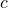, and classify those observations with a fitted probability aboveas positive and those at or below it as negative. For this particular cut-off, we can estimate the sensitivity by the proportion of observations withwhich have a predicted probability above, and similarly we can estimate specificity by the proportion ofobservations with a predicted probability at or below.

If we increase the cut-point, fewer observations will be predicted as positive. This will mean that fewer of theobservations will be predicted as positive (reduced sensitivity), but more of theobservations will be predicted as negative (increased specificity). In picking the cut-point, there is thus an intrinsic trade off between sensitivity and specificity.

The receiver operating characteristic (ROC) curve
Now we come to the ROC curve, which is simply a plot of the values of sensitivity against one minus specificity, as the value of the cut-pointis increased from 0 through to 1: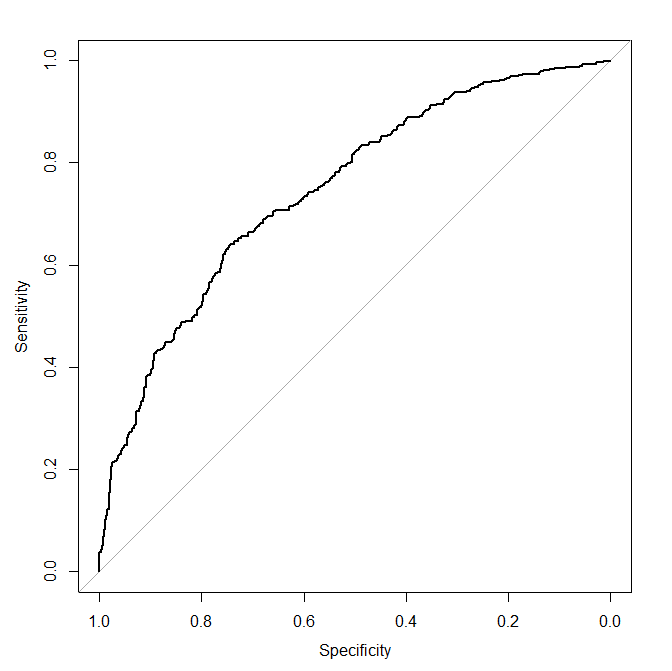A model with high discrimination ability will have high sensitivity and specificity simultaneously, leading to an ROC curve which goes close to the top left corner of the plot. A model with no discrimination ability will have an ROC curve which is the 45 degree diagonal line.

Plotting the ROC curve in R
There are a number of packages in R for creating ROC curves. The one I’ve used here is the pROC package. First, let’s simulate a dataset with one predictor x:

set.seed(63126)
n <- 1000
x <- rnorm(n)
pr <- exp(x)/(1+exp(x))
y <- 1*(runif(n) < pr)
mod <- glm(y~x, family="binomial")


Next we extract from the fitted model object the vector of fitted probabilities:

predpr <- predict(mod,type=c("response"))

We now load the pROC package, and use the roc function to generate an roc object. The basic syntax is to specify a regression type equation with the response y on the left hand side and the object containing the fitted probabilities on the right hand side:

library(pROC)
roccurve <- roc(y ~ predpr)

The roc object can then be plotted using

plot(roccurve)


which gives us the ROC plot (see previously shown plot). Note that here because our logistic regression model only included one covariate, the ROC curve would look exactly the same if we had used roc(y ~ x), i.e. we needn't have fitted the logistic regression model. This is because with just one covariate the fitted probabilities are a monotonic function of the only covariate. However in general (i.e. with more than one covariate in the model), this won't be the case.

Previously we said that a model with good discrimination ability, the ROC curve will go close to the top left corner. To check this with a simulation, we will re-simulate the data, increasing the log odds ratio from 1 to 5:

set.seed(63126)
n <- 1000
x <- rnorm(n)
pr <- exp(5*x)/(1+exp(5*x))
y <- 1*(runif(n) < pr)
mod <- glm(y~x, family="binomial")

predpr <- predict(mod,type=c("response"))

roccurve <- roc(y ~ predpr)
plot(roccurve)


which gives

Now let's run the simulation one more time but where the variable x is in fact independent of y. To do this we simply modify the line generating the probability vector pr to

pr <- exp(0*x)/(1+exp(0*x))

which gives the following ROC curve

Area under the ROC curve
A popular way of summarizing the discrimination ability of a model is to report the area under the ROC curve. We have seen that a model with discrimination ability has an ROC curve which goes closer to the top left hand corner of the plot, whereas a model with no discrimination ability has an ROC curve close to a 45 degree line. Thus the area under the curve ranges from 1, corresponding to perfect discrimination, to 0.5, corresponding to a model with no discrimination ability. The area under the ROC curve is also sometimes referred to as the c-statistic (c for concordance).

The area under the estimated ROC curve (AUC) is reported when we plot the ROC curve in R's Console. We can also obtain the AUC using

auc(roccurve)


I'll return to the topics of confidence interval estimation for the estimated AUC and adjusting for optimism in later posts.

For more information on the pROC package, I'd suggest taking a look at this paper, published in the open access journal BMC Bioinformatics.

Interpretation of the area under the ROC curve
Although it is not obvious from its definition, the area under the ROC curve (AUC) has a somewhat appealing interpretation. It turns out that the AUC is the probability that if you were to take a random pair of observations, one withand one with, the observation withhas a higher predicted probability than the other. The AUC thus gives the probability that the model correctly ranks such pairs of observations.

In the biomedical context of risk prediction modelling, the AUC has been criticized by some. In the risk prediction context, individuals have their risk of developing (for example) coronary heart disease over the next 10 years predicted. Thus a measure of discrimination which examines the predicted probability of pairs of individuals, one withand one with, does not really match the prospective risk prediction setting, where we do not have such pairs.

For more on risk prediction, and other approaches to assessing the discrimination of logistic (and other) regression models, I'd recommend looking at Steyerberg's Clinical Prediction Models book, an (open access) article published in Epidemiology, and Harrell's Regression Modeling Strategies' book.

### 20 thoughts on “Area under the ROC curve – assessing discrimination in logistic regression”

1.The cut-point was called ‘p’ and then referred to as ‘c’.

•Many thanks Anvesh! I have corrected this now.

2.Hello John,

Do we have to check for good calibration before plotting ROC curve and conducting DeLong test?
What are other ways to check calibration other than Hosmer-Lemeshow test?

Thanks

•Hi Bila

One alternative to graphically assess calibration is to plot the binary outcome against the model predicted probability of success, with a lowess smoother. If the model is well calibrated, the lowess smoother line should follow a 45 degree line, i.e. observed risk matches predicted risk.

Jonathan

3.Jonathan, Excellent posts on binary classifiers, thanks. However, should the ROC chart not be a plot of sensitivity vs 1-specificity (True Positive Rate vs False Positive Rate)? Your text in the paragraph under the section heading “The receiver operating characteristic curve (ROC) curve” states this, but the axis label reads specificity. I ask because the open access article you have provided a link for states that AUC and concordance are the same for an ROC plot of TPR vs 1-FPR (which, if I have understood the concept properly, should be TPR vs FPR).

•Thanks Rao. The pRoc package labels the x-axis as specificity, but then puts a reverse axis there – the axis runs from 1 to 0. I think the intention is that is easier than a standard axis which would be labeled 1-sp, but I think it’s quite likely that people may not spot the reverse axis also!

4.Hi Jonathan, again to be sure about the ROC plot: You are saying that only x-axis label is different, but the plot is correct. Am I right?
In that case, one can use xlab=”” command to put 1-specificity on the x axis. Am I right?

•Hi Mitra. Yes, the package authors I think thought that a good default behaviour is to use a reverse x-axis scale, so that the x-axis is specificity, rather than 1-specificity.

To have it label the x-axis in the ‘traditional’ way, i.e. 1-specificity, you can specify the legacy.axes=TRUE option when calling the plot function. See http://cran.r-project.org/web/packages/pROC/pROC.pdf for more info.

5.Thanks for the post on ROC curve
Can we draw a Roc curve to assess the goodness of fit in GLM poisson with robust variance estimate?
I am working with a prediction model on adherence to arv treatment using Glm poisson. I will appreciate any help.

•It is not obvious to me how one could use the ROC curve with a Poisson GLM, since the outcome in a Poisson model is a count, rather than binary, and so it is unclear how you would define sensitivity and specificity. There are however alternative goodness of fit tests for Poisson regression. One of the best sources of information on this is the book Regression Analysis of Count Data Book by Cameron and Trivedi.

•Many Thanks Jonathan for your feedback. The think is that I have a binary outcome wich is poor adherence to ARV treatment after 6 months(Yes/No). So I am using the GLM poisson regression model with robust variance estimate to estimate a relative risk or risk ratio. I previously used the log binomial model as recommended when the outcone is rare nut it failed to converge either in R and Stata.
Now that I have a final model I wanted to assess the discriminative ability and whether the model fits the observed data.
I bought the book Generalized linear Model and Extensions ( Hardin and Hilbe third edition) but what I realised is that they only give use measure such as R, AIC, BIC. I think such measure are only when one want to compare two nested models in GLM models.
Someone has also advice me to use the linktest in Stata. Many thanks for helping

•Hi again Salam.

I see, so your outcome is in fact binary (although, as you explained, you are using Poisson GLM to estimate risk ratios). In this case I think you ought to be able to use ROC, and perhaps the area under it, to assess discrimination. You can simply take the linear predictor from your fitted Poisson model, and use this as your ‘diagnostic test’. In Stata you could use the roctab command to calculate the AUC, with refvar being the subject’s true (binary) status and the classvar their linear predictor from the Poisson model.

Best wishes
Jonathan

6.This is a very useful website-thanks for setting it up!

I’m new to AUC/ROC analyses and I see there are different methods and variations upon you can try -parametric, semi-parametric and non-parametric. I’ve been going through some key books/papers etc. trying to find a simple description of how you could decide (either in advance or posthoc) which method(s) are most appropriate given the characteristics of the data you’re working with, but have not had much success. I understand the difference between parametric/non-parametric tests in other contexts, but can’t quite make the connection between how you would decide which AUC method is most appropriate for any given analysis. If you know of a reference that might help to clear this up that would be great!

•Hi Ron

This paper (http://www.ncbi.nlm.nih.gov/pmc/articles/PMC2774909/), focuses on Stata commands for estimating ROC curves, but has a little discussion on parametric versus non-parametric approaches. Advantages of parametric approaches are that they give you a smooth estimates ROC curve that will be more precisely estimated, provided the parametric assumptions made are appropriate for the data at hand. In general I think unless you want to model how discrimination varies with covariates, the non-parametric approach is the most popular, since one does not have to worry about checking parametric assumptions. I have a recollection of a paper comparing empirically parametric, semi-parametric and non-parametric approaches, but at present can’t remember the title/authors etc. Sorry.

Best wishes
Jonathan

7.Hi Jonathan!

Thank you for this very interesting post. I got one question regarding the link between the AUC and the probability of correctly ranking two randomly observations (one from the diseased and one from the non-diseased) that you explained in the section “interpretation of the area under the roc curve”. The point is that I did not manage to mathematically “demonstrate” that area under the curve “sensitivity vs 1-specificity” is similar to calculating the rate of concordant pairs (p(Xi) > p(Xj)).

Please could you provide a kind of proof.

Best regards

•Hi Oliver. Please see for a proof of this result.

Best wishes, Jonathan

•Thank you Jonathan. I red this but actually I did not understand the step from the simple integral to the double ones.
Oliver

8.Hi, if the AUC is below 0.5, is there something wrong with the statistics? I ran the AUC and ROC analyses in SPSS and it turns out the AUC is around .280, which is really low. However, sensitivity, specificity and predictive values are all alright, all higher than 0.6.

•9.